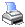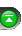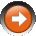﻿ Moving Average - Exponential (EMA)
 NinjaScript > Language Reference > Indicator Methods > Moving Average - Exponential (EMA)Description

The exponential moving average is but one type of a moving average. In a simple moving average, all price data has an equal weight in the computation of the average with the oldest value removed as each new value is added. In the exponential moving average equation the most recent market action is assigned greater importance as the average is calculated. The oldest pricing data in the exponential moving average is however never removed.

Syntax

 EMA(int period) EMA(IDataSeries input, int period)   Returns default value EMA(int period)[int barsAgo] EMA(IDataSeries input, int period)[int barsAgo]

Return Value

double; Accessing this method via an index value [int barsAgo] returns the indicator value of the referenced bar.

Parameters

 input Indicator source data (?) period Number of bars used in the calculation

Examples

 // Prints the current value of a 20 period EMA using default price type double value = EMA(20); Print("The current EMA value is " + value.ToString());   // Prints the current value of a 20 period EMA using high price type double value = EMA(High, 20); Print("The current EMA value is " + value.ToString());

Source Code

You can view this indicator method source code by selecting the menu Tools > Edit NinjaScript > Indicator within the NinjaTrader Control Center window.